# Texas Go Math Grade 1 Lesson 2.5 Answer Key Order Numbers on an Open Number Line

Refer to our Texas Go Math Grade 1 Answer Key Pdf to score good marks in the exams. Test yourself by practicing the problems from Texas Go Math Grade 1 Lesson 2.5 Answer Key Order Numbers on an Open Number Line.

## Texas Go Math Grade 1 Lesson 2.5 Answer Key Order Numbers on an Open Number Line

Esential Question
How can you order numbers using a number line?
Explanation:
When ordering numbers, we first start by comparing two numbers at a time. You can do this by either arranging the numbers in ascending or descending order.

Explore
Draw lines to show where the numbers belong on the number line.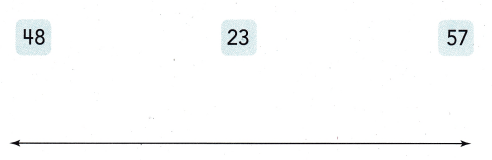For The Teacher

• Read the following problem. Mikka wants to place these three numbers on the number line. Where do the numbers belong?
Explanation:Numbers placed according to Least to greatest.

Math Talk
Mathematical processes
Now Mikka wants to place 55 on the number line. Between which two numbers does 55 belong? Explain.

Model and Draw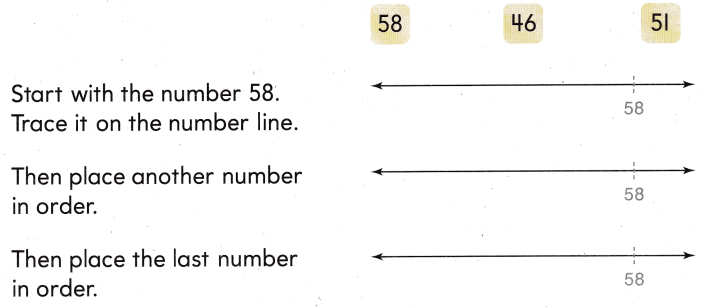Use place value. Write the numbers in order.
Explanation: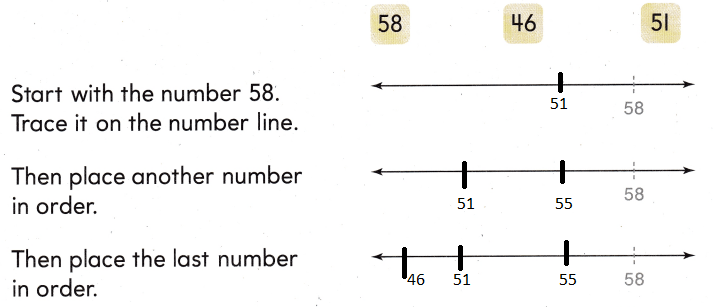Explanation: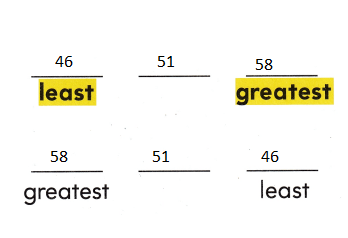Think
Compare the tens. Then compare the ones.

Share and Show

Use place value and the number line to order numbers. Then write the numbers in order.

Question 1.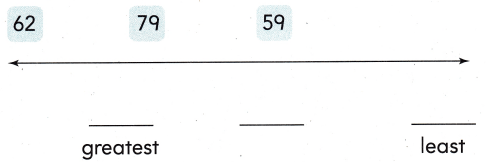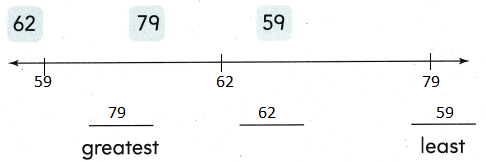Explanation:
Numbers are arranged according to least to greatest
and written greatest to least

Problem Solving

Use place value and the number line to order numbers. Then write the numbers in order.

Question 2.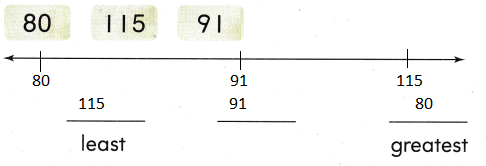Explanation:
Numbers are arranged according to least to greatest
and written least to greatest

Solve. Write or draw to explain.

Question 3.
H.O.T. Ava saved 105 cans. Martin saved 96 cans, Brandon saved III cans, and Shellie saved 92. Write the numbers of cans saved in order from least to greatest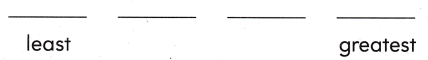Explanation:
Numbers are arranged according to least to greatest
and written  least to greatest

Question 4.
Multi-Step The vet places dogs in order by their tag numbers. The brown dog is tag number 120, the black dog is 109, and the white dog is 119. Write the colors of the dogs in tag number order from greatest to least.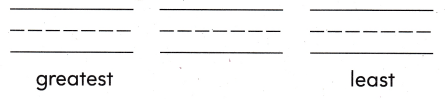Explanation:
120, 119 , 109
he colors of the dogs in tag number order from greatest to least

Question 5.
Multi-Step Drew, Matt, and Bradley play football. Their numbers are 15, 12 and 23. Which shows the numbers in order from greatest to least?
(A) 23, 12, 15
(B) 12, 23, 15
(C) 23, 15, 12
Explanation:
Greatest to least are
23, 15, 12

Question 6.
Which shows numbers in order from least to greatest?
(A) 31, 47, 39
(B) 29, 44, 49
(C) 59, 19, 32
Explanation:
29, 44, 49 shows numbers in order from least to greatest

Question 7.
Apply Which shows numbers in order from greatest to least?
(A) 54, 76, 18
(B) 113, 102, 29
(C) 36, 119, 63
Explanation:
113, 102, 29 shows numbers in order from greatest to least

Question 8.
Texas Test Prep Use place value. Which shows the numbers in order from east to greatest?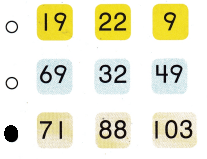Explanation:
71 , 88, 103 shows the numbers in order from least to greatest

Take Home Activity

• Give your child the numbers 48, 57, and 102. Have your child use a number line to order the numbers from least to greatest.
48, 57, and 102. are in   order the numbers from least to greatest.
Explanation: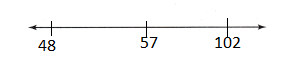### Texas Go Math Grade 1 Lesson 2.5 Homework and Practice Answer Key

Use place value and the number line to order numbers. Then write the numbers in order.

Question 1.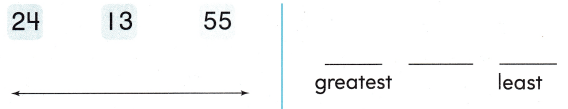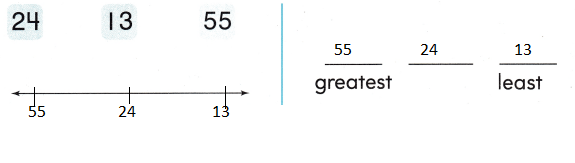Explanation:
55 , 24, 13 shows the numbers in order from greatest to least

Question 2.Explanation:
112, 104, 93 shows the numbers in order from  least to greatest

Question 3.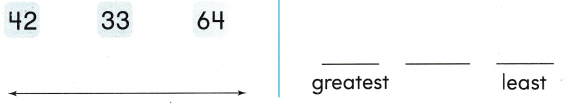Explanation:
64, 42, 33 shows the numbers in order from greatest to least

Problem Solving

Write or draw to explain.

Question 4.
Tony saved 63 pennies. Jill saved 103 pennies and Ed saved 33 pennies. Write the number of pennies saved in
order from least to greatest.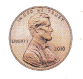Explanation:
33 , 63 , 103 the number of pennies saved in
order from least to greatest.

Texas Test Prep

Lesson Check

Question 5.
Lily got scores of 92, 84, and 100. Which shows the numbers in order from greatest to least?
(A) 100, 92, 84
(B) 84, 100, 92
(C) 100, 84, 92
Explanation:
100, 92, 84 shows the numbers in order from greatest to least

Question 6.
Rabbit ate 14 carrots. Robin ate 21 worms. Bear ate 13 fish. Which shows the numbers in order from least to greatest?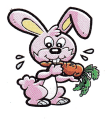(A) 14, 21, 13
(B) 21, 14, 13
(C) 13, 14, 21
Explanation:
13, 14, 21 shows the numbers in order from least to greatest

Question 7.
Which shows the numbers in order from greatest to least?
(A) 107, 55, 83
(B) 94, 93, 10
(C) 59, 14, 102